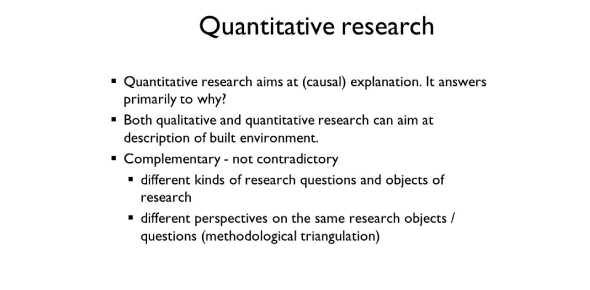# 7031 Quantitative Research-pre-test

7 Questions | Total Attempts: 673SettingsThis pretest is not for a grade. It is only for instructor use.

Related Topics
• 1.
According to the text, which of the following terms refers to an individual unit of study that isnot a human being?
• A.

Case

• B.

Subject

• C.

Participant

• D.

Sample

• 2.
When the value of a trait being measure is the same for all cases, we refer to this value as a
• A.

Unit

• B.

Continuous variable

• C.

Parameter

• D.

Constant

• 3.
Despite the fact that most of the statistics discussed in the text are inappropriate for ordinal data, _____ coefficient can be used to measure the degree of relationship between two sets of ranks.
• A.

Pearson rho

• B.

Spearman rho

• C.

Z. test

• D.

Kappa

• 4.
For which of the following scales must we assume that the distance between two adjacent scores is not equal to the distance between two other adjacent scores?
• A.

Nominal

• B.

Ordinal

• C.

Proportional

• D.

Ratio

• 5.
______ variables are variables whose values theoretically could fall anywhere between adjacent scale units.
• A.

Continuous

• B.

Discrete

• C.

Dichotomous

• D.

Nominal

• 6.
A ______ lists each observed score, along with the number of cases falling at each score. a. normal distribution
• A.

Normal distribution

• B.

Frequency table

• C.

Percentile table

• D.

Score Interval

• 7.
Which way of presenting data can be described as a hybrid between a table and a graph?
• A.

Histogram

• B.

Realitive frequency table

• C.